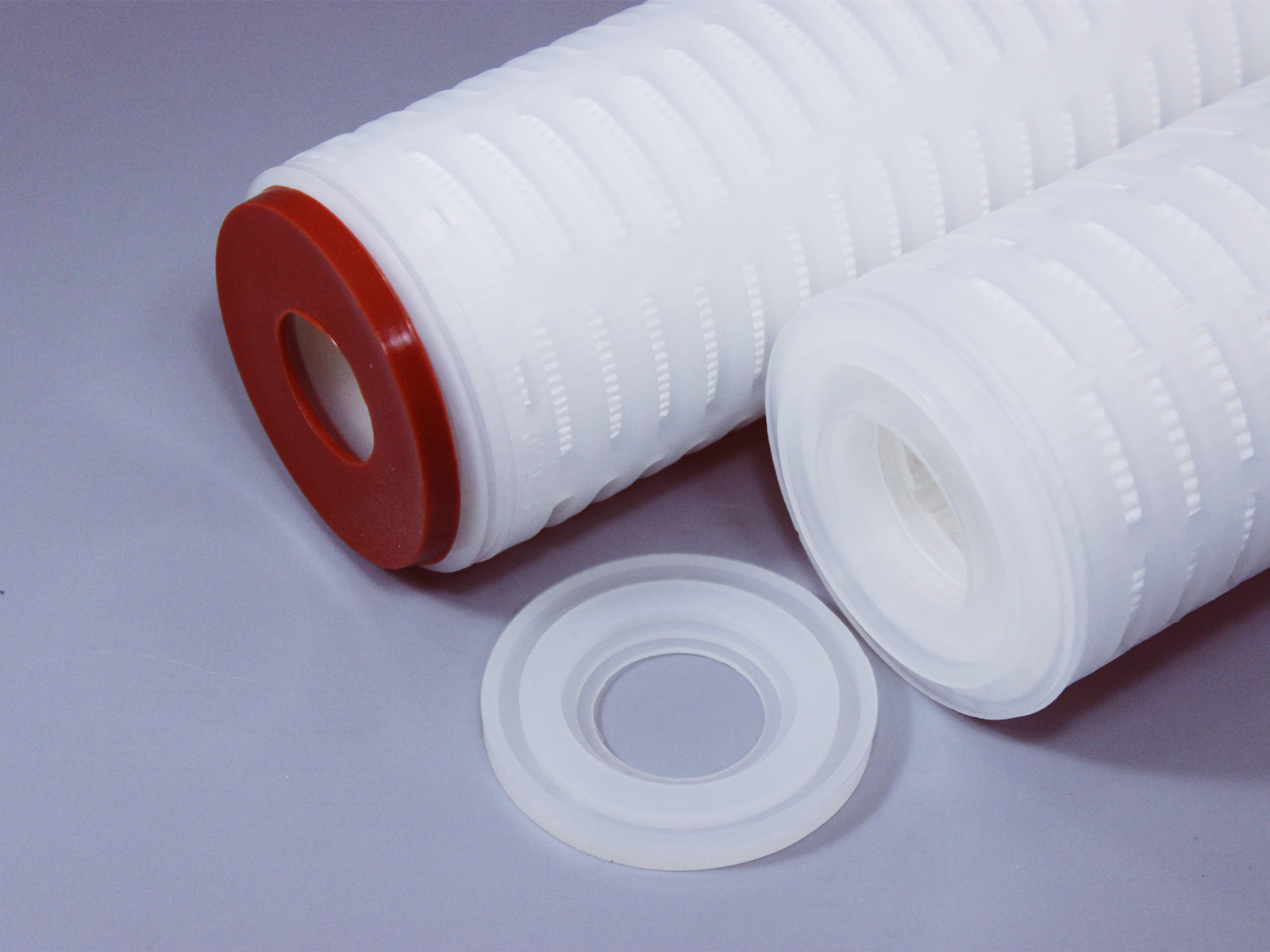# 公司产品# PPA高精度聚丙烯滤芯

--截留效率>99.8%的聚丙烯滤芯

PPA系列滤芯，采用双层梯度聚丙烯膜，具有高纳污量，长寿命，高效率的特性。同时支撑导流层选用美国进口长纤材料，保证无纤维脱落。双层过滤使整支滤芯的过滤效果可达99.8%以上，是一支高精度聚丙烯滤芯。

• 产品概述
• 规格参数
• 产品选型

PPA高精度聚丙烯滤芯

--截留效率>99.8%的聚丙烯滤芯

PPA系列滤芯，采用双层梯度聚丙烯膜，具有高纳污量，长寿命，高效率的特性。同时支撑导流层选用美国进口长纤材料，保证无纤维脱落。双层过滤使整支滤芯的过滤效果可达99.8%以上，是一支高精度聚丙烯滤芯。

RO（反渗透）预过滤，保安过滤

 酸 R 苯 NR 甲基乙基酮 R 碱 R 甲苯 NR 甲基异丁基酮 R 酯 R 二甲苯 NR 二氯甲烷 LR 醇 R 芳香烃 NR 二氯化乙烯 LR 丙酮 R 环己酮 R 全氯乙烯 LR

R: 表示能耐受此化学试剂     LR: 表示能有限度的耐受此化学试剂            NR: 不能耐受此化学试剂

PH值：1-14

 PPA40E0500S PPA 长度 接口 精度 密封材质 05=5英寸 A=平压 0010=0.1μm 1000=10μm S=硅橡胶 10=10英寸 B=222/翅片 0022=0.22μm 3000=30μm M=三元乙丙 20=20英寸 C=226/翅片 0045=0.45μm 5000=50μm N=丁腈橡胶 30=30英寸 D=222/平封 0100=1μm 7000=70μm V=氟橡胶 40=40英寸 E=226/平封 0300=3μm 100H=100μm T=铁氟龙包氟 F=215/平封 0500=5μm

# 相关推荐X